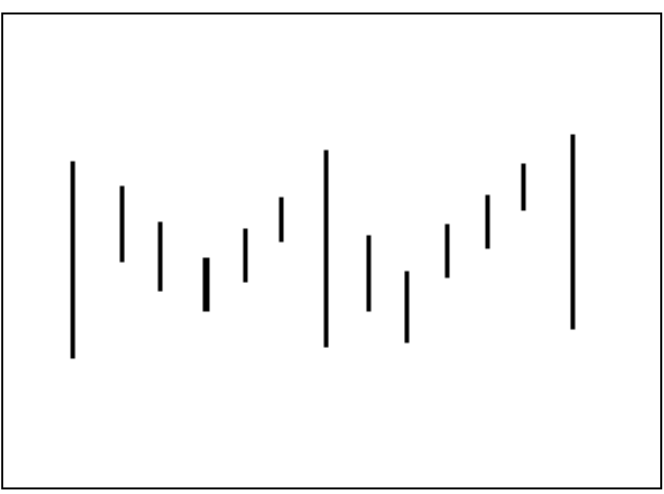Search
•arque algo trader

Volatility Cluster Strategy

Updated: May 30, 2020

With Nifty, Sensex volatility at an all time high, we are all wondering when and how to grab the opportunity provided by this unique situation.

While studying volatility breakouts we often assume that volatility of one bar will be higher per every x number of bars of normal volatility.

The pattern would look like something given below:So, on an average, for every six periods of normal volatility, there is one period of high volatility.

However, in reality, periods of high volatility usually appear in clusters or groups ie. one period of high volatility will usually be followed by another of either the same or slightly varying magnitude. This happens because the underlying event that causes one high volatility period will usually not subside within just one period. Its effects will be witnessed over several periods.

Mathematically speaking, when an extraordinary (indiscrete) event occurs (for eg. Influence of a news story over a stock price), we represent this in indiscrete terms (bars).

The high volatility periods will look something like the below chart:Our Vol Clustering strategy will identify for high volatility in the below 2 ways:

1. As periods in which the range increases significantly from its average.

2. As periods in which the difference between current close to previous close increases significantly from the average difference between 2 consecutive closes.

Also, we will only identify movements which are more than 2 standard deviations greater than the average movement. In this case, stop loss orders will be placed both above and below the market to enter either long or short, depending on which way the market breaks out.

To determine the entry price for either direction, follow below steps:

1. Calculate three-bar average of the range

2. Add it to the close

3. Subtract it (three bar average) from the close

To calculate the three bar average, we will leave out the bar which shows volatility spike, since the range of this bar is greater than the average. Instead, we will use the three bar average of the range of one bar before the high volatility bar.

Exit will be at the first open which meets the profit target.

The trade set up will be as follows:

1. Calculate the difference between the close of the current bar and the close of the previous bar.

2. Calculate the 18-bar average of the difference between two consecutive closes.

3. To this average, add two standard deviations of the difference of closes over 18 bars.

4. Calculate 18-bar average of the range and to this average, add two standard deviations of the range over the last 18 bars.

Long entry:

a) Compare the difference between two consecutive closes to the standard deviation calculation from the setup. If the difference is greater, place a buy stop loss at the close plus the three-bar average of the range (of one bar ago).

b) Compare the range to the standard deviation calculation from the setup. If the range is greater, place a buy stop at the close plus the three-bar average of the range (of one bar ago).

Short Entries:

a) Compare the difference between the two consecutive closes to the standard deviation calculation from the setup. If the difference is greater, place a sell stop loss at the close minus the three-bar average of the range (of one bar ago).

b) Compare the range to the standard deviation calculation from the setup. If the range is greater, place a sell stop at the close minus the three-bar average of the range (of one bar ago).Long and Short Exits

Exit at the first profitable open. This is calculated by comparing the open with the entry price (and accounting for a desired profit expressed in points and commission).

Do you have any further optimization ideas for this strategy?? Leave your thoughts in the comments below.

Do you want to backtest & optimize your trading strategy? Write to us at contact@arque.tech